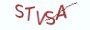# 2.2.2 算法实现

```# __author__ = 薯条老师

"""
:param number:待转换的十进制数
"""
radix_prefix = {2: "0b", 8: "0", 10: "", 16: "0x"}
try:
except KeyError:
number = -number if number < 0 else number

# 在itoa内部定义一个递归的闭包函数decimal_to_binary
def decimal_to_binary(n):
# 仅需一行代码即可实现十进制转二进制
return str(n) if n <= 1 else decimal_to_binary(n // radix) + str(n % radix)

return prefix + decimal_to_binary(number)

if __name__ == "__main__":
print(itoa(15))```

# 2.2.3 最具实力的小班培训

`(1) Python后端工程师高薪就业班，月薪11K-18K，免费领取课程大纲(2) Python爬虫工程师高薪就业班，年薪十五万，免费领取课程大纲(3) Java后端开发工程师高薪就业班，月薪11K-20K, 免费领取课程大纲(4) Python大数据分析，量化投资就业班，月薪12K-25K,免费领取课程大纲`## 注册账号